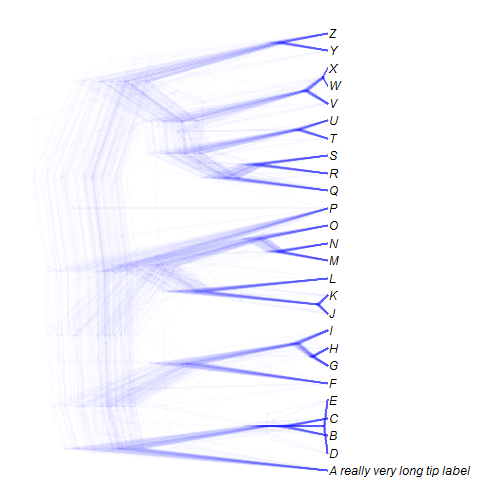## Tuesday, September 26, 2017

### Possible solution for functions that use `plot.phylo` or `plotTree` argument internally for the problem of blank space in knitted markdown files

An R-sig-phylo subscriber today posted the following:

“I am writing a function that calls `plot.phylo` in the ape package with the option `plot=FALSE`. I then do some calculations with the output (the calculated coordinates), and then make another call to `plot.phylo` with `plot=TRUE`. If I do this, then the `plot=FALSE` option creates a blank plot, which is the result that is clearly documented in the help pages. However, I do not want this blank plot, because I am saving the result (the second call to `plot.phylo`) to a pdf, and so the pdf has a blank page before the plot I want. And since Iâ€™m calling this in a function, I can't "wait” for the second plot (a similar problem occurs if you run it in a knitr document) Is there a way to get the output from `plot.phylo` with all of the coordinates, etc. without having to have the blank plot? (I do not want an option to “add” the plot to the blank plot, if it exists, because I am doing these calculations so that in my next call I can change the `x.lim` options so the tree only takes up a fraction of the plot, so I need to reset the `par`, etc., and not just draw on the original coordinates set up by the blank plot).“

Although this question may seem a little esoteric, it actually applies to some functions of phytools that use the similar `plotTree(...,plot=FALSE)` or `plotSimmap(...,plot=FALSE)` calls internally, to obtain certain values from the plot but without plotting, and then plot subsequently to this. The result looks completely fine in an interactive R session - but results in a big white space in knitted R markdown (or a blank sheet in a PDF). For instance, the function `densityTree` suffers this ailment, e.g.:

``````library(phytools)
for(i in 1:length(trees))
trees[[i]]\$tip.label[which(trees[[i]]\$tip.label=="A")]<-
"A really very long tip label"
show.axis=FALSE)
``````The reason `densityTree` does this is because it wants to find the plotting dimensions of the longest of our set of trees before plotting any of the trees so that it can use a x scale that works for all trees. This is more complicated than just the total length of the tree because R needs to open a plotting device to permit the function to figure out how much space to leave for the tip labels. This is why I substituted the very long tip label for the label A in this example to ensure that when we make any changes, we are still getting that complicated spacing right!

Rafael Maia shared the very simple solution as follows:

"I ran into a similar problem recently (with another package) and a simple solution that worked for me is to call `par(new=TRUE)` before your `plot=TRUE` plot.”

The documentation of `par` says the following about argument `new`:

`new`: logical, defaulting to `FALSE`. If set to `TRUE`, the next high-level plotting command (actually `plot.new`) should not clean the frame before drawing as if it were on a new device. It is an error (ignored with a warning) to try to use `new = TRUE` on a device that does not currently contain a high-level plot.

Now let's try to insert this solution into the code of `densityTree` & see what happens:

``````## function to be used internally
rescaleTree<-function(tree,scale){
tree\$edge.length<-tree\$edge.length/max(nodeHeights(tree))*scale
tree
}
## function to plot a posterior density of trees (e.g., densiTree in phangorn)
## written by Liam J. Revell 2016, 2017
densityTree<-function(trees,colors="blue",alpha=NULL,method="plotTree",
fix.depth=FALSE,use.edge.length=TRUE,compute.consensus=TRUE,
N<-length(trees)
if(any(sapply(trees,function(x) is.null(x\$edge.length))))
use.edge.length<-FALSE
if(!use.edge.length){
trees<-lapply(trees,compute.brlen)
class(trees)<-"multiPhylo"
}
h<-sapply(trees,function(x) max(nodeHeights(x)))
if(fix.depth){
if(method=="plotTree"){
trees<-lapply(trees,rescaleTree,mean(h))
class(trees)<-"multiPhylo"
} else if(method=="plotSimmap"){
trees<-rescaleSimmap(trees,depth=mean(h))
}
h<-sapply(trees,function(x) max(nodeHeights(x)))
}
tips<-setNames(1:Ntip(trees[]),
if(compute.consensus) untangle(consensus(trees),
else trees[]\$tip.label)
if(is.null(alpha)) alpha<-max(c(1/N,0.01))
args<-list(...)
args\$direction<-"leftwards"
args\$tips<-tips
if(is.null(args\$nodes)) args\$nodes<-"inner"
if(is.null(args\$mar)) args\$mar<-if(show.axis) c(4.1,1.1,1.1,1.1) else rep(1.1,4)
if(is.null(args\$ftype)) args\$ftype<-"i"
plotTree(trees[[which(h==max(h))]],direction="leftwards",mar=args\$mar,
plot=FALSE)
par(new=TRUE) ## Maia solution here
args\$xlim<-get("last_plot.phylo",envir=.PlotPhyloEnv)\$x.lim[2:1]
if(method=="plotTree"){
args\$color<-make.transparent(colors,alpha)
for(i in 1:length(trees)){
args\$tree<-trees[[i]]
do.call(plotTree,args)
if(i==1){
if(show.axis) axis(1)
args\$ftype<-"off"
}
}
} else if(method=="plotSimmap"){
states<-sort(unique(as.vector(mapped.states(trees))))
if(length(colors)!=length(states)){
colors<-setNames(c("grey",palette()[2:length(states)]),
states)
}
colors<-sapply(colors,make.transparent,alpha=alpha)
args\$colors<-colors
for(i in 1:length(trees)){
args\$tree<-trees[[i]]
do.call(plotSimmap,args)
if(i==1){
if(show.axis) axis(1)
args\$ftype<-"off"
}
}
}
rf<-multiRF(trees,quiet=TRUE)
mds<-cmdscale(rf,k=1)[,1]
trees<-trees[order(mds)]
h<-h[order(mds)]
args\$ylim<-c(0,Ntip(trees[])+1)
plotTree(trees[[which(h==max(h))]],direction="leftwards",mar=args\$mar,
ylim=args\$ylim,plot=FALSE)
par(new=TRUE) ## also here
args\$xlim<-get("last_plot.phylo",envir=.PlotPhyloEnv)\$x.lim[2:1]
colors<-sapply(rainbow(n=length(trees)),make.transparent,alpha=alpha)
ftype<-args\$ftype
for(i in 1:length(trees)){
y.shift<-(i-median(1:length(trees)))/length(trees)/2
args\$tree<-trees[[i]]
args\$tips<-tips+y.shift
args\$color<-colors[i]
args\$ftype<-if(i==floor(median(1:length(trees)))) ftype else "off"
do.call(plotTree,args)
if(i==1){
if(show.axis) axis(1)
args\$ftype<-"off"
}
}
}
}
``````

Try it:

``````densityTree(trees,type="cladogram",nodes="intermediate",
show.axis=FALSE)
``````Cool. Works!